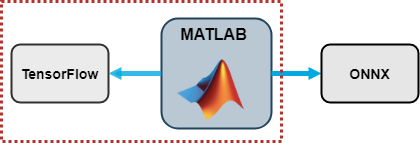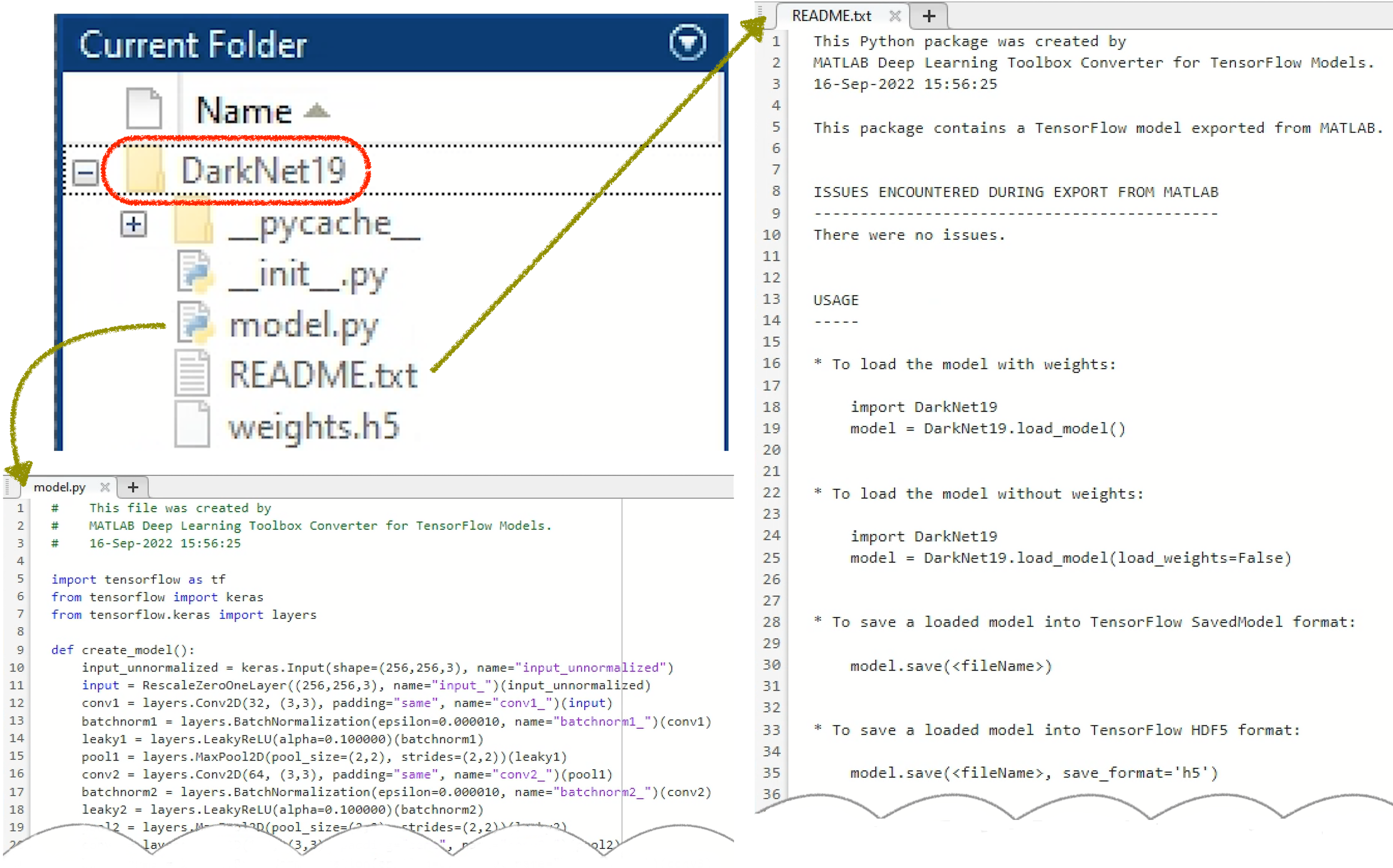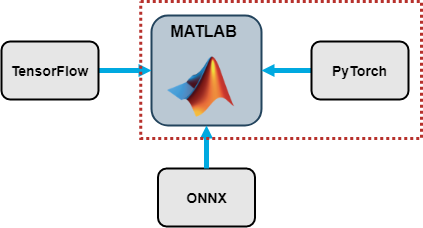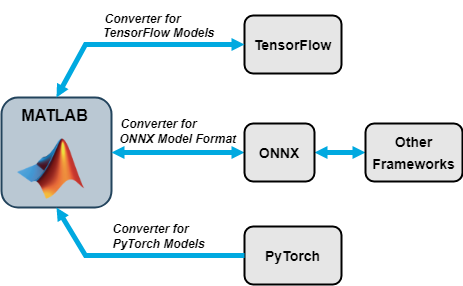# What’s New in Interoperability with TensorFlow and PyTorch

For deep learning, MATLAB allows users to create and train models in MATLAB or leverage models trained in open source via model conversion. Prior to MATLAB R2022b, support for model conversion included: import from and export to ONNX™, and import from TensorFlow™. We are excited to share that as of MATLAB R2022b, users can now export models to TensorFlow as Python® code and can import models from PyTorch® (starting with support for image classification).

Export to TensorFlowThe support package Deep Learning Toolbox Converter for TensorFlow Models just added the capability to export from MATLAB to TensorFlow, by using the exportNetworkToTensorFlow function. There are many reasons to be excited about the new exportNetworkToTensorFlow function:
 You can export deep learning networks and layer graphs (e.g., convolutional, LSTM) directly to TensorFlow. It’s easy to export a network, load it as a TensorFlow model, and use the exported model for prediction. For a relevant example, see Export Network to TensorFlow and Classify Image. It’s easy to export an untrained layer graph, load it as a TensorFlow model, and train the exported model. For a relevant example, see Export Untrained Layer Graph to TensorFlow. You can save the exported model in any standard TensorFlow format, such as SavedModel or HDF5 format, and share it with your colleagues who work in TensorFlow. Many MATLAB layers can be converted to TensorFlow layers. For a full list of the layers, see Layers Supported for Export to TensorFlow.
Here I am showing the basic workflow of how to export a deep learning network to TensorFlow, load it as a TensorFlow model, and save it in SavedModel format.
 MATLAB Code: Load a pretrained network. The Pretrained Deep Neural Networks documentation page shows you all options of how to get a pretrained network. You can alternatively create your own network. net = darknet19; Export the network net to TensorFlow. The exportNetworkToTensorFlow function saves the TensorFlow model in the Python package DarkNet19.  exportNetworkToTensorFlow(net,"DarkNet19")
The DarkNet19 package contains four files:
• The _init_.py file, which defines the DarkNet19 folder as a regular Python package.
• The model.py file, which contains the code that defines the untrained TensorFlow-Keras model.
• The README.txt file, which provides instructions on how to load the TensorFlow model and save it in HDF5 or SavedModel format.
• The weights.h5 file which contains the model weights in HDF5 format.Figure: The exported TensorFlow model is saved in the regular Python package DarkNet19.

 Python Code: Load the exported TensorFlow model from the DarkNet19 package. import DarkNet19 model = DarkNet19.load_model() Save the exported model in the SavedModel format. model.save("DarkNet19_savedmodel")

Import from PyTorchIn R2022b we introduced the Deep Learning Toolbox Converter for PyTorch Models support package. This initial release supports importing image classification models. Support for other model types will be added in future releases. Use the importNetworkFromPyTorch function to import a PyTorch model. Make sure that the PyTorch model that you are importing is pretrained and traced. I am showing you here how to import an image classification model from PyTorch and initialize it.
 Python Code: Load a pretrained image classification model from the TorchVision library. import torch from torchvision import models model = models.mnasnet1_0(pretrained=True) Trace the PyTorch model. For more information on how to trace a PyTorch model, go to Torch documentation: Tracing a function. X = torch.rand(1,3,224,224) traced_model = torch.jit.trace(model.forward,X)  Save the PyTorch model. traced_model.save("traced_mnasnet1_0.pt") 
 MATLAB Code: Import the PyTorch model into MATLAB by using the importNetworkTFromPyTorch function. The function imports the model as an uninitialized dlnetwork object without an input layer. net = importNetworkFromPyTorch("traced_mnasnet1_0.pt");  Specify the input size of the imported network and create an image input layer. Then, add the image input layer to the imported network and initialize the network by using the addInputLayer function (also new in R2022b). InputSize = [224 224 3]; InputLayer = imageInputLayer(InputSize,Normalization="none"); net = addInputLayer(net,InputLayer,Initialize=true); 
You might have noticed in the above code that the input dimensions in PyTorch and MATLAB have a different order. For more information, see Input Dimension Ordering for Deep Learning Platforms. For more details on how to import a PyTorch model, how to initialize the imported model, and how to perform workflows (such as prediction and training) on the imported model, see the Examples of the importNetworkTFromPyTorch documentation page.

Interoperability Capabilities SummaryThe interoperability support packages allow you to connect Deep Learning Toolbox with TensorFlow, Pytorch, and ONNX. Use the import and export functions to access models available in open-source repositories and collaborate with colleagues who work in other deep learning frameworks. More information:
|